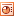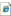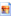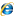# All Other NumberRecurring Decimals to Fractions

Here's eight numbers to practise converting from recurring decimal to fractions. Covers all key recurring-patterns that students need to be aware of. Includes answers. PDF here.Numeracy Checkup

Sets of questions on various basic numeracy methods. Includes fractions of, percentages of, simplifying linear algebraic expressions, solving simple equations, decimal addition plus several more. 'How to...' document for students to learn independently.PFF to fnd HCF & LCM

Pupils use prime factor trees & prime factor form to find the highest common factor and lowest common multiple of two numbers. Answers included.Number Lines

Printable sheet of various number lines for students to use, count on, cut out, stick in... whatever you choose!Number Line

A good number line with various options and a zoom facilityExcel file of numberlines for general use, with several different starting values and increment options.Special Ratios

An real-life investigation based activity to encourage students to 'discover' the golden ration, pi and root 2, in relation to their common everyday occurences. Golden ratio, golden ratio in hair, golden ratio in mona lisa, golden ratio in parthenon, golden ratio in flower.Relationships, PDF here

A number machine, algebra, graph, sentence, sequence and input/output table for students to investigate... and hopefully come to the conclusion that all represent the same relationship in different ways. Suggestions for ways to investigate are on slide 4. To extend this rich open task students could create their own set of cards. Lesson plan. A more structured version (PDF here) for students requiring more support.

A subsequent activity is for students to complete the number machine, table, sentence and graph from the algebra. Prompt cards (PDF here)for this activity with lesson plan.Five to Eight

Four questions all relating to the 5:8 ratio; percentage increase, miles to kilometres, ratio and enlargement. Designed to highlight the similarities between such areas of Mathematics. Another version of this worksheet.Mathematical Relations?

A development of the 'five to eight' idea, to emphasize the similarities and relationships between apparently unconnected areas of mathematics. Pupils spend 10 minutes working on each of the 4 sections before concluding that they've been using same techniques to master enlargement, percentage increase, measurement and ratio.Missing Multiplications

Pupils use knowledge of times tables to complete the missing spaces on the tables. Note that digits 2-9 are used only once along top row & LHS column.Roman Numerals

A rich maths resource on investigating numbers written in Roman numerals. Converting to and from, sequences of Roman numerals and patterns withn them. Lots of mathematical reasoning. Answers and links included.Sexagesimal

A rich maths resource on investigating numbers written in Sexagesimal, or Base 60. Converting to and from and calculating with. Lots of mathematical reasoning. Answers and links included.Binary

A rich maths resource on investigating numbers written in Binary. Converting to and from and calculating with. A large version of the 'Hold up cards'. Lots of mathematical reasoning. Answers and links included. For lower ability students use some grids for students to write summations of powers of two and then convert into binary (there is a very brief lesson plan included here also).Comparing Number Systems

Intended for use after studying the above activities on number systems to make explicit the understanding acheived. Most probably the answers suggested aren't definitive and certainly should create discussion and reasoning.Number Patterns Introduction

Match up cards with picture sequences on the front and, scambled, letters for words & numbers on the reverse. We've split the set into two halves: counting, doubling, evens & odds all match up together and then triangle, square and Fibonacci to extend learning afterwards.Excel file of numberlines for general use, with several different starting values and increment options.Mathematics of a Total Solar Eclipse

A good 'similar triangles' activity to explain the shadow of a total solar eclipse. Harder examples of the main activity.Lesson planBulletpoints for lessonMore structured task for less experienced pupilsSupporting presentationSOHO image of SunEclipse mapSOHO websiteHow Many Records?

Another mathematical conundrum. Adapted from a rather boring example, this time its DJ's Roni Size & Norman Cook arguing about who has the most records. Can you help them figure it out?! (There's a hint on the second page if you need it).The Missing Pound

Brilliant little trick / riddle. Read it and get your pupils, friends at the pub, enemies etc to try to figure it out. Email me if you need a hint!Maths Puzzle

A good deduction based puzzle for pupils of many ages.Maths Puzzle 2

A shakedown of understanding of column addition methods, BIDMAS, substitution, factors, multiples... leading to an SMSC consideration of the relative frequencies of letters used in the english language.

 Smartboard file starterLesson planKLO'sGraph of letter frequencies by frequencyGraph of letter frequencies by letterFind the Code

Find the code from the given clues using algebra or otherwise.Decimals Rule

How to add, subtract, multiply & divide decimals. Crib sheet that I typed for my Yr8 group. Follow it and you can't go wrong!Million

Use your knowledge of Trial & Improvement Techniques to try to 'Win a Million!'